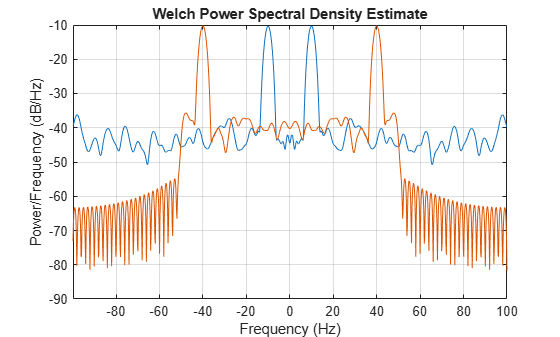modulate

Modulation for communications simulation

Description

y = modulate(x,fc,fs) modulates the real message signal x with a carrier frequency fc and sample rate fs. If x is a matrix, the modulated signal is computed independently for each column and stored in the corresponding column of y.

[y,t] = modulate(x,fc,fs) also returns the internal time vector t.

example

[___] = modulate(x,fc,fs,method) modulates the real message signal using the modulation technique specified by method. You can use these inputs with either of the previous output syntaxes.

example

[___] = modulate(x,fc,fs,method,opt) uses the additional options specified in opt for some modulation methods.

Examples

collapse all

Generate a 10 Hz sinusoidal signal sampled at a rate of 200 Hz for 1 second. Embed the sinusoid in white Gaussian noise of variance 0.01.

fs = 200;
t = 0:1/fs:1;
x = sin(2*pi*10*t) + randn(size(t))/10;

Single-sideband amplitude modulate the signal with a carrier frequency of 50 Hz. Compute and display the new Welch's power spectral density estimates.

y = modulate(x,50,fs,'amssb');

pwelch([x;y]',hamming(100),80,1024,fs,'centered')Generate two sinusoidal signals frequencies of 10 Hz and 20 Hz, sampled at a rate of 200 Hz for 1 second. Embed the sinusoids in white Gaussian noise of variance 0.01.

fs = 200;
t = 0:1/fs:1;
i = sin(2*pi*10*t) + randn(size(t))/10;
q = sin(2*pi*20*t) + randn(size(t))/10;

Create a quadrature amplitude modulated signal from signals i and q using a carrier frequency of 70 Hz. Compute the Welch power spectral density estimates of the original and modulated sequences. Use a 100-sample Hamming window with 80 samples of overlap. Specify an FFT length of 1024.

y = modulate(i,70,fs,'qam',q);

pwelch([i;q;y]',hamming(100),80,1024,fs,'centered')Input Arguments

collapse all

Message signal, specified as a real vector or matrix.

Example: sin(2*pi*25*[0:(1/200):1])

Carrier frequency used to modulate the message signal, specified as a real positive scalar.

Sample rate, specified as a real positive scalar.

Method of modulation used, specified as one of:

• amdsb-sc or am — Amplitude modulation, double sideband, suppressed carrier. Multiplies x by a sinusoid of frequency fc.

y = x.*cos(2*pi*fc*t)

• amdsb-tc — Amplitude modulation, double sideband, transmitted carrier. Subtracts scalar opt from x and multiplies the result by a sinusoid of frequency fc.

y = (x-opt).*cos(2*pi*fc*t)

If you do not specify the opt parameter, modulate uses a default of min(min(x)) so that the message signal (x-opt) is entirely nonnegative and has a minimum value of 0.

• amssb — Amplitude modulation, single sideband. Multiplies x by a sinusoid of frequency fc and adds the result to the Hilbert transform of x multiplied by a phase-shifted sinusoid of frequency fc.

y = x.*cos(2*pi*fc*t)+imag(hilbert(x)).*sin(2*pi*fc*t)

This effectively removes the upper sideband.

• fm — Frequency modulation. Creates a sinusoid with instantaneous frequency that varies with the message signal x.

y = cos(2*pi*fc*t + opt*cumsum(x))

cumsum is a rectangular approximation of the integral of x. modulate uses opt as the constant of frequency modulation. If you do not specify the opt parameter, modulate uses a default of opt = (fc/fs)*2*pi/(max(max(x))) so the maximum frequency excursion from fc is fc Hz.

• pm — Phase modulation. Creates a sinusoid of frequency fc whose phase varies with the message signal x.

y = cos(2*pi*fc*t + opt*x)

modulate uses opt as the constant of phase modulation. If you do not specify the opt parameter, modulate uses a default of opt = pi/(max(max(x))) so the maximum phase excursion is π radians.

• pwm — Pulse-width modulation. Creates a pulse-width modulated signal from the pulse widths in x. The elements of x must be between 0 and 1, specifying the width of each pulse in fractions of a period. The pulses start at the beginning of each period, that is, they are left justified. modulate(x,fc,fs,'pwm','centered') yields pulses centered at the beginning of each period. The length of y is length(x)*fs/fc.

• ppm — Pulse-position modulation. Creates a pulse-position modulated signal from the pulse positions in x. The elements of x must be between 0 and 1, specifying the left edge of each pulse in fractions of a period. opt is a scalar between 0 and 1 that specifies the length of each pulse in fractions of a period. The default for opt is 0.1. The length of y is length(x)*fs/fc.

• qam— Quadrature amplitude modulation. Creates a quadrature amplitude modulated signal from signals x and opt.

y = x.*cos(2*pi*fc*t) + opt.*sin(2*pi*fc*t)

The input argument opt must be the same size as x.

Optional input, specified for some methods. Refer to method for more details on how to use opt.

Output Arguments

collapse all

Modulated message signal, returned as a real vector or matrix. Except for the methods pwm and ppm, y is the same size as x.

Internal time array used by modulate in its computations, specified as a real vector.